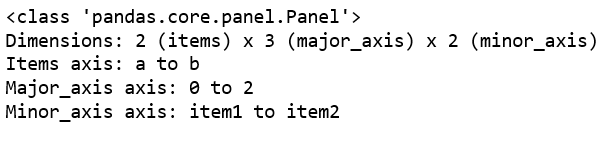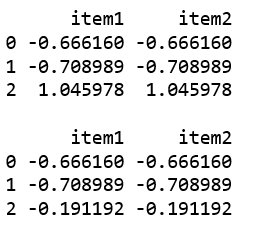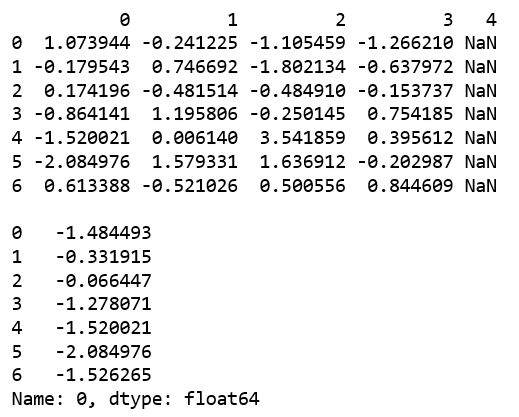Related Articles

# Python | Pandas Panel.clip_upper()

• Last Updated : 01 Jan, 2019

In Pandas, Panel is a very important container for three-dimensional data. The names for the 3 axes are intended to give some semantic meaning to describing operations involving panel data and, in particular, econometric analysis of panel data.

`Panel.clip_upper()` function is used to return copy of input with values above given value(s) truncated.

Syntax: Panel.clip_upper(threshold, axis=None, inplace=False)

Parameters:
threshold : float or array_like
axis : Align object with threshold along the given axis.
inplace : Whether to perform the operation in place on the data

Returns: same type as input.

Creating a Panel :

 `# importing pandas module ``import` `pandas as pd ``import` `numpy as np`` ` `df1 ``=` `pd.DataFrame({``'a'``: [``'Geeks'``, ``'For'``, ``'geeks'``], ``                    ``'b'``: np.random.randn(``3``)})``                     ` `data ``=` `{``'item1'``:df1, ``'item2'``:df1}`` ` `# creating Panel ``panel ``=` `pd.Panel.from_dict(data, orient ``=``'minor'``)``print``(panel, ``"\n"``)`

Output:Code #1: Using clip_upper()

 `# importing pandas module ``import` `pandas as pd ``import` `numpy as np`` ` `df1 ``=` `pd.DataFrame({``'a'``: [``'Geeks'``, ``'For'``, ``'geeks'``], ``                    ``'b'``: np.random.randn(``3``)})``                     ` `data ``=` `{``'item1'``:df1, ``'item2'``:df1}`` ` `# creating Panel ``panel ``=` `pd.Panel.from_dict(data, orient ``=``'minor'``)``print``(panel, ``"\n"``)``print``(panel[``'b'``], ``'\n'``)`` ` ` ` `df2 ``=` `pd.DataFrame({``'b'``: np.random.randn(``5``)})``print``(panel[``'b'``].clip_upper(df2[``'b'``], axis ``=` `0``))`

Output:Code #2: Using clip_upper()

 `# creating an empty panel``import` `pandas as pd``import` `numpy as np`` ` `data ``=` `{``'Item1'` `: pd.DataFrame(np.random.randn(``7``, ``4``)), ``        ``'Item2'` `: pd.DataFrame(np.random.randn(``4``, ``5``))}``         ` `pen ``=` `pd.Panel(data)``print``(pen[``'Item1'``], ``'\n'``)`` ` `p ``=` `pen[``'Item1'``][``0``].clip_upper(np.random.randn(``7``))``print``(p)`

Output:Attention geek! Strengthen your foundations with the Python Programming Foundation Course and learn the basics.

To begin with, your interview preparations Enhance your Data Structures concepts with the Python DS Course. And to begin with your Machine Learning Journey, join the Machine Learning – Basic Level Course

My Personal Notes arrow_drop_up